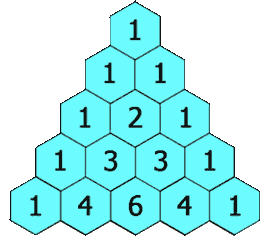## Generating Rows of Pascal's Triangle

Pascal's triangle is a triangular arrangement of numbers where the $n^{th}$ row gives the coefficients of $(x+y)^n$. Consequently, $$\begin{array}{c} (x+y)^0 = 1\\ (x+y)^1 = x + y\\ (x+y)^2 = x^2 + 2xy + y^2\\ (x+y)^3 = x^3 + 3x^2 y + 3xy^2 + y^3\\ (x+y)^4 = x^4 + 4x^3 y + 6x^2 y^2 + 4x y^3 + y^4\\ \end{array}$$ reveals rows 0 through 4 of Pascal's triangle to be:There are simpler ways to generate Pascal's triangle, however. The classic approach is to notice that the left and right sides will always consist of 1's, while each interior value is simply the sum of the two values directly above it -- as the below graphic demonstrates.Another approach is to generate each row in the following manner: Suppose you wish to generate the 6th row (i.e., the one that corresponds to $(x+y)^6$). We know it must begin with a one, so we write that down. $$1$$ Then, we multiply this value by a fraction, formed by putting the row number in the numerator, and a 1 in the denominator. The product is the second element in the row. Since in this example, we are interested in row 6, we have: $$1 \times \frac{6}{1} = 6$$ We create a new fraction by decreasing the numerator by 1 and increasing the denominator by 1. The product of this new fraction and our previous value gives the third element in the row. $$6 \times \frac{5}{2} = 15$$ Continuing in this way, decrementing the numerator, incrementing the denominator, and then multiplying the new fraction by the previous value (until we get a 1) yields the rest of the elements in the row: $$\begin{array}{rcl} 15 \times \frac{4}{3} &=& 20\\ 20 \times \frac{3}{4} &=& 15\\ 15 \times \frac{2}{5} &=& 6\\ 6 \times \frac{1}{6} &=& 1\\ \end{array}$$ So here, the 6th row of Pascal's triangle should be: 1, 6, 15, 20, 15, 6, 1.